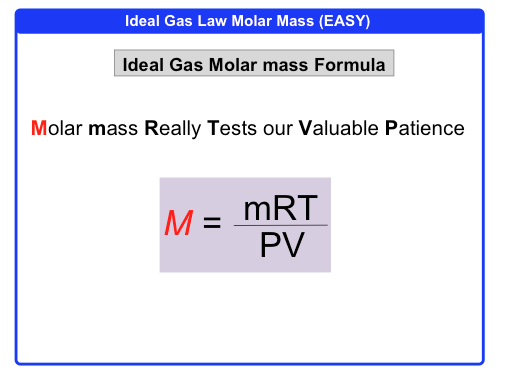Clutch Prep is now a part of Pearson

# The Ideal Gas Law: Molar Mass

See all sections
Sections
Pressure Units
The Ideal Gas Law
The Ideal Gas Law Derivations
The Ideal Gas Law Applications
Chemistry Gas Laws
Chemistry Gas Laws: Combined Gas Law
Mole Fraction
Partial Pressure
The Ideal Gas Law: Molar Mass
The Ideal Gas Law: Density
Gas Stoichiometry
Standard Temperature and Pressure
Effusion
Root Mean Square Speed
Kinetic Energy of Gases
Maxwell-Boltzmann Distribution
Velocity Distributions
Kinetic Molecular Theory
Van der Waals Equation
Boyle's Law (IGNORE)
Charles Law (IGNORE)
Ideal Gas Law (IGNORE)

The Ideal Gas Law can be further extended to find the molar mass of a gas.

###### The Ideal Gas Law (Molar Mass)

Concept #1: Molar Mass

Example #1: Calculate the molar mass of a gas if 2.50 g occupies 0.995 L at 715 torr and 40 ºC.

Concept #2: Ideal Gas Law Molar Mass (EASY)Concept #3: Ideal Gas Law Molar Mass (HARD)

Example #2: An unknown gas with mass of 0.1727 g is placed into a 125-mL flask. If its pressure is 0.833 atm at 20.0ºC, what is the identity of the gas?

Practice: To identify a homonuclear diatomic gas, a chemist weighted an evacuated flask with a volume of 3.9 L then filled it with the gas at a pressure of 2.00 atm and 29.0 ºC. The chemist then re-weighted the flask and recorded the difference in mass as 8.81 g. Identify the gas.

a) H2

b) N2

c) Cl2

d) F2

e) O2

Practice: What is the molecular formula of a compound that contains 39.0% carbon, 16.0% hydrogen, and 45.0% nitrogen, if 0.1576 g of the compound occupies 125 mL with a pressure of 0.9820 atm at 295.15 K?